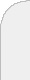inicioir al DIIbuscarmodels and algorithms for traffic engineering in ip networks  Most data networks nowadays are based on the Internet Protocol (IP) and employ shortest path routing protocols to control the flow of the data packets. With such a protocol, all end-to-end traffic streams are routed along shortest paths with respect to some administrative routing weights. Dimensioning and routing planning are extremely difficult for such networks, because the end-to-end routing paths can be controlled only indirectly via the routing weights and only jointly for all traffic demands. It is not possible to configure the end-to-end paths for the demands individually. Additional difficulties arise if the communication demands must be sent unsplit through the network - a requirement that is often imposed to ensure tracability of end-to-end traffic flows and to prevent package reordering in practice. In this case, the lengths must be chosen such that the shortest paths are uniquely determined for all communication demands. In this talk, we consider the problem of finding an unsplittable shortest path routing routing (USPR) that minimizes the maximum link congestion in a communication network: Given a capacitated digraph $D=(V,A)$ and traffic demands $d_{s,t}$, $(s,t)\in K\subseteq V^2$, the task is to find routing weights $\lambda_a\in\mathbb{Z}_+$, $a\in A$, such that the shortest $(s,t)$-path is unique for each $(s,t)\in K$ and the maximum arc congestion (flow/capacity ratio) is minimal. We discuss the relation of USPR to other routing schemes, review some results concerning the approximability, and present two solution approaches for the congestion minimization problem. Our first approach relies on a mixed integer linear programming formulation based on an independence system characterization of the path sets that form USPRs. Our second approach decomposes the problem into a number of simple shortest path computations via Lagrangian relaxation. We report on computational results for the German research.